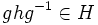# Central implies normal

This article gives the statement and possibly, proof, of an implication relation between two subgroup properties. That is, it states that every subgroup satisfying the first subgroup property (i.e., central subgroup) must also satisfy the second subgroup property (i.e., normal subgroup)
View all subgroup property implications | View all subgroup property non-implications

## Statement

Suppose$H$ is a subgroup of$G$ that is a central subgroup of$G$, i.e.,$H$ is contained in the center$Z(G)$ of$G$. Then,$H$ is a normal subgroup of$G$.

## Related properties

### Intermediate properties

Property Proof that central subgroup implies it Proof that it does not imply central Proof that it implies normal subgroup Proof that normal subgroup does not imply it
central factor central implies central factor central factor not implies central central factor implies normal normal not implies central factor
hereditarily normal subgroup central implies hereditarily normal hereditarily normal not implies central (immediate) (via transitively normal)
transitively normal subgroup (via hereditarily normal) (via hereditarily normal) (immediate) normality is not transitive
abelian normal subgroup central implies abelian and central implies normal abelian normal not implies central (immediate) every group is normal in itself, combined with existence of non-abelian groups.

## Related facts

### Converse

The converse is not true in general: normal not implies central.

However, some versions are true:

## Proof

### Proof using coset definition of normality

Given: A group$G$, a central subgroup$H$ of$G$.

To prove:$gH = Hg$ for all$g \in G$ (the cosets definition of normality).

Proof: Since$H$ is central, this means that$gh = hg$ for all$g \in G$,$h \in H$. Thus, for a fixed$g$, the sets$gH = \{ gh: h \in H \}$ and$Hg = \{ hg : h \in H \}$ are equal, because each$gh$ equals the corresponding$hg$.

### Proof using conjugation definition of normality

Given: A group$G$, a central subgroup$H$ of$G$.

To prove: For all$g \in G$ and$h \in H$, we have$ghg^{-1} \in H$.

Proof: Since$h$ is central, we have, by definition, that$gh = hg$ for all$g \in G$. Multiplying both sides on the right by$g^{-1}$, we obtain that$ghg^{-1} = h$ for all$g \in G$. Since$h \in H$ by assumption, and$ghg^{-1} = h$, we obtain that$ghg^{-1} = h$.

### Proof using commutator definition of normality

Given: A group$G$, a central subgroup$H$ of$G$.

To prove: For all$g \in G$,$h \in H$, we have$ghg^{-1}h^{-1} \in H$.

Proof: Since$h \in H$ we have$gh = hg$ by definition. Multiplying both sides by$g^{-1}h^{-1}$ on the right, we get$ghg^{-1}h^{-1} = e$ (i.e., it is the identity element). Since any subgroup contains the identity element,$e \in H$, so $-1}{h^{-1$ .

### Proof using union of conjugacy classes definition of normality

Given: A group$G$, a central subgroup$H$ of$G$.

To prove:$H$ is a union of conjugacy classes in$G$.

Proof: Every element of the center of$G$ forms a conjugacy class of size 1. Since$H$ comprises only central elements, it is the union of these singleton conjugacy classes.